Space over an algebra

(diff) ← Older revision | Latest revision (diff) | Newer revision → (diff)

A space with a differential-geometric structure whose points can be provided with coordinates from some algebra. In most cases the algebra is assumed to be associative with identity, and sometimes alternative with identity (cf. Associative rings and algebras; Alternative rings and algebras).

To construct a large class of spaces over an algebra one can start with a module over the algebra, whose definition can be obtained from that of a vector space over a skew-field by replacing the skew-field by an associative algebra with identity (cf. , ). By relating to the elements of the module, called vectors, new elements, called points, related to the vectors by the same axioms as the points of an affine space are related to its vectors, one obtains an affine space over an associative algebra with identity. The affine transformations in the affine space over an algebra have coordinate representation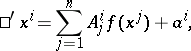whereis a continuous algebra automorphism. The-dimensional affine space over an algebra, of rankover a certain field, has a unique model (representation) in the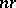-dimensional affine space over the same field. In this model, every pointof the affine space over the algebra is mapped to a point of the-dimensional affine space over the field considered whose coordinates are the expansion coefficients of the coordinates ofin the basis elements of the algebra. If the basis elements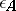,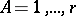, of the algebra are related by structure equations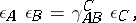where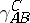are the structure constants of the algebra, then each basis elementcorresponds in the model to the linear transformation with matrix(*)

whereidentical-dimensional blocks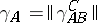occupy the principal diagonal. In affine spaces over algebras one can specify a Hermitian metric (Euclidean and pseudo-Euclidean), while in the case of commutative algebras even a quadratic (Euclidean and pseudo-Euclidean) metric can be given. To do this one defines in a unital module a scalar vector product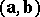, in the first case with the property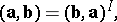whereis an involutory anti-automorphism (an involution) of the algebra, and in the second case with the propertyThe scalar square of a vector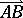determines a metric invariant of the pair of points. The motions of Euclidean and pseudo-Euclidean spaces are the affine transformations preserving the scalar vector product. Replacing in the definition of elliptic and hyperbolic spaces over an algebra the scalar vector product by a scalar vector product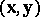for which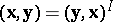or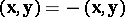, one obtains Hermitian, or quadratic symplectic, spaces over an algebra.

The variety of one-dimensional submodules of an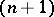-dimensional unital module over an algebrais called the-dimensional projective space over; its points are the one-dimensional submodules, while the projective coordinates of a point are the coordinates of any vector generating the corresponding one-dimensional submodule. In a projective space over an algebra one defines collineations and correlations as in a projective space over a field (cf. Collineation; Correlation). In projective coordinates, collineations have the form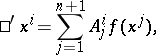whereis a continuous algebra automorphism, and correlations have the formwhereis a continuous algebra anti-automorphism, and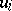are projective hyperplane coordinates. The introduction of a scalar vector product in a unital module makes it possible to define in a projective space constructed by means of this module Hermitian, or in the case of a commutative algebra — quadratic elliptic and hyperbolic, metrics. The scalar products of vectorsof the corresponding submodules determine metric invariants of the points of these spaces by means of the cross ratioIfis a real number, then the invariantfor whichis called the distance between the corresponding points (cf. ). Projective, elliptic, hyperbolic, and symplectic spaces over real simple algebras (e.g., the algebras of real, complex or quaternion matrices) have the property that their fundamental groups are simple Lie groups of an infinite series. Euclidean, pseudo-Euclidean, quasi-elliptic, quasi-hyperbolic, and quasi-symplectic spaces over the same algebras have the property that their fundamental groups are quasi-simple Lie groups of the same series (cf. ); the same property is shared by projective, elliptic, hyperbolic, and symplectic spaces over semi-simple algebras, to which also belongs the algebra of dual numbers.

Projective and Hermitian (elliptic and parabolic) planes over alternative algebras are defined in a more involved manner. Their fundamental groups are simple or quasi-simple Lie groups of certain exceptional types.

How to Cite This Entry:
Space over an algebra. Encyclopedia of Mathematics. URL: http://encyclopediaofmath.org/index.php?title=Space_over_an_algebra&oldid=13356
This article was adapted from an original article by B.A. Rozen'feldA.P. Shirokov (originator), which appeared in Encyclopedia of Mathematics - ISBN 1402006098. See original article